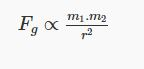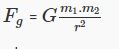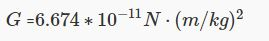Universal law of gravitation

This is published on 5 july 1687 According to Newton’s Universal Law of Gravitation, the force exerted between two objects, by each other is given by the following relation. Law of universal gravitation states that any two bodies in the universal attract each other with a force that is direct proportional to the product of their masses and inversely proportional to the square of the distance between them .this is a general physics law from empirical observations by what Isaac Newton called introduction.• Where Fg is gravitational between two bodies
• m1 is the mass of an object
• m2 is the mass of second object
• r is the distance between the centres of two bodies .

• The actual force exerted between two bodies can be given by the following equationWhere G is the universal gravitational constant with a valueG here is an empirical constant of proportionality

Difference between G and g in gravitation

G is universal gravitation constant whose approximately value is 6.673×10−11N. And "g" is accelaration due to gravity whose value is 9.8 meter per second2 .